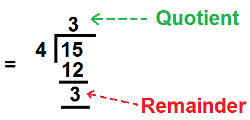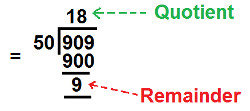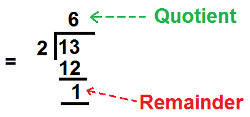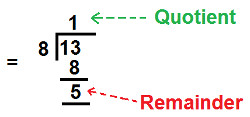Arithmetic
Arithmetic Progression
Associative Property
Averages
Brackets
Closure Property
Commutative Property
Conversion of Measurement Units
Cube Root
Decimal
Distributivity of Multiplication over Addition
Divisibility Principles
Equality
Exponents
Factors
Fractions
Fundamental Operations
H.C.F / G.C.D
Integers
L.C.M
Multiples
Multiplicative Identity
Multiplicative Inverse
Numbers
Percentages
Profit and Loss
Ratio and Proportion
Simple Interest
Square Root
Unitary Method
Algebra
Cartesian System
Order Relation
Polynomials
Probability
Standard Identities & their applications
Transpose
Geometry
Basic Geometrical Terms
Circle
Curves
Angles
Define Line, Line Segment and Rays
Non-Collinear Points
Parallelogram
Rectangle
Rhombus
Square
Three dimensional object
Trapezium
Triangle
Trigonometry
Trigonometry Ratios
Data-Handling
Arithmetic Mean
Frequency Distribution Table
Graphs
Median
Mode
Range

Videos
Solved Problems
Home >> Decimal >> Decimal to Mixed Fraction >> Examples

## Convert Decimal to Mixed Fraction : Solved Examples

 Decimal Point Place Value of digits in decimals Expanded Form of Decimal Read Decimals Decimal Places Decimal to Fraction Decimal to Mixed Fraction Decimal to Lowest Fractional form Decimal into Percentage Like Decimals Unlike Decimals Difference Like & Unlike Decimals Comparing or Ordering Decimals Ascending Order of Decimals Descending Order of Decimals Multiplication of Decimals Division of Decimals

Convert the following decimals into Mixed fraction

A) 3.75
B) 1.90
C) 18.18
D) 6.5
E) 1.625
A) 3.75

Solution: Follow the steps of conversion:
Step 1: Numerator of fraction is equal to decimal (without the decimal point) and we get: Numerator = 375

Step 2 : Find number of Decimal Place in the given decimal
Decimals Places = two (i.e. digit 7 and 5)

Step 3: Denominator of fraction is equal to 1 followed by as many number of zero as there are decimal place.

As calculated in step 2, there are two decimal place, so we will write two zeros next to digit 1 and we get:

Denominator = 100

 Now, we know that: Fraction = NumeratorDenominator

Put the values of numerator and denominator from step 1 & Step 3 and we get:

Fraction = 375
100

Now we will convert this fraction into lowest form

Step 4: Find HCF of numerator and denominator of fraction (calculated above)

Fraction = 375
100

HCF of 375 and 100 = 25

Step 5: Divide both numerator and denominator by HCF and we get:
 Fraction = 375 ÷ 25100 ÷ 25

Solve the above division expression and we get:

Fraction = 15
4

Now to convert above calculated fraction into mixed fraction, follow the below steps:

Step 6: Divide Numerator by denominator of above calculated fraction i.e.

 154Step 7: Quotient will be Whole Number Part of Mixed Fraction.

so, Quotient = Whole number Part = 3 (calculated above)

Step 8: Remainder will be now be the Numerator of Mixed Fraction.

so, Remainder = Numerator = 3 (calculated above)

Step 9: Denominator remains same, as calculated in step 5.

So, denominator = 4 and we get:

Mixed Fraction = 3 3
4

Solution: Follow the steps of conversion:
Step 1: Numerator of fraction is equal to decimal (without the decimal point) and we get:

Numerator = 1.90

Step 2 : Find number of Decimal Place in the given decimal

Decimals Places = two (i.e. digit 9 and 0)

Step 3: Denominator of fraction is equal to 1 followed by as many number of zero as there are decimal place.

As calculated in step 2, there are two decimal places, so we will write two zeros next to digit 1 and we get:

Denominator = 100

 Now, we know that: Fraction = NumeratorDenominator

Put the values of numerator and denominator from step 1 & Step 3 and we get:

Fraction = 190
100

Now we will convert this fraction into lowest form

Step 4: Find HCF of numerator and denominator of fraction (calculated above)

Fraction = 190
100

HCF of 190 and 100 = 10

Step 5: Divide both numerator and denominator by HCF and we get:
 Fraction = 190 ÷ 10100 ÷ 10

Solve the above division expression and we get:

Fraction = 19
10

Now to convert above calculated fraction into mixed fraction, follow the below steps:

Step 6: Divide Numerator by denominator of above calculated fraction i.e.

 1910Step 7: Quotient will be Whole Number Part of Mixed Fraction.

so, Quotient = Whole number Part = 1 (calculated above)

Step 8: Remainder will be now be the Numerator of Mixed Fraction.

so, Remainder = Numerator = 9 (calculated above)

Step 9: Denominator remains same, as calculated in step 5.

So, denominator = 10 and we get:

Mixed Fraction = 1 9
10

C) 18.18

Solution: Follow the steps of conversion:

Step 1: Numerator of fraction is equal to decimal (without the decimal point) and we get:

Numerator = 18.18

Step 2 : Find number of Decimal Place in the given decimal

Decimals Places = two (i.e. digit 1 and 8)

Step 3: Denominator of fraction is equal to 1 followed by as many number of zero as there are decimal place.

As calculated in step 2, there are two decimal places, so we will write two zeros next to digit 1 and we get:

Denominator = 100

 Now, we know that: Fraction = NumeratorDenominator

Put the values of numerator and denominator from step 1 & Step 3 and we get:

Fraction = 1818
100

Now we will convert this fraction into lowest form

Step 4: Find HCF of numerator and denominator of fraction (calculated above)

Fraction = 1818
100

HCF of 1818 and 100 = 2

Step 5: Divide both numerator and denominator by HCF and we get:
 Fraction = 1818 ÷ 2100 ÷ 2

Solve the above division expression and we get:

Fraction = 909
50

Now to convert above calculated fraction into mixed fraction, follow the below steps:

Step 6: Divide Numerator by denominator of above calculated fraction i.e.

 90950Step 7: Quotient will be Whole Number Part of Mixed Fraction.

so, Quotient = Whole number Part = 18 (calculated above)

Step 8: Remainder will be now be the Numerator of Mixed Fraction.

so, Remainder = Numerator = 9 (calculated above)

Step 9: Denominator remains same, as calculated in step 5.

So, denominator = 50 and we get:

Mixed Fraction = 18 9
50

D) 6.5

Solution: Follow the steps of conversion:

Step 1: Numerator of fraction is equal to decimal (without the decimal point) and we get:

Numerator = 65

Step 2 : Find number of Decimal Place in the given decimal

Decimals Places = one only (i.e. digit 5)

Step 3: Denominator of fraction is equal to 1 followed by as many number of zero as there are decimal place.

As calculated in step 2, there is only one decimal place, so we will write one zero next to digit 1 and we get:

Denominator = 10

 Now, we know that: Fraction = NumeratorDenominator

Put the values of numerator and denominator from step 1 & Step 3 and we get:

Fraction = 65
10

Now we will convert this fraction into lowest form

Step 4: Find HCF of numerator and denominator of fraction (calculated above)

Fraction = 65
10

HCF of 65 and 10 = 5

Step 5: Divide both numerator and denominator by HCF and we get:
 Fraction = 65 ÷ 510 ÷ 5

Solve the above division expression and we get:

Fraction = 13
2

Now to convert above calculated fraction into mixed fraction, follow the below steps:

Step 6: Divide Numerator by denominator of above calculated fraction i.e.

 132Step 7: Quotient will be Whole Number Part of Mixed Fraction.

so, Quotient = Whole number Part = 6 (calculated above)

Step 8: Remainder will be now be the Numerator of Mixed Fraction.

so, Remainder = Numerator = 1 (calculated above)

Step 9: Denominator remains same, as calculated in step 5.

So, denominator = 2 and we get:

Mixed Fraction = 6 1
2

E) 1.625

Solution: Follow the steps of conversion:

Step 1: Numerator of fraction is equal to decimal (without the decimal point) and we get:

Numerator = 1625

Step 2 : Find number of Decimal Place in the given decimal

Decimals Places = three (i.e. digit 6, 2 and 5)

Step 3: Denominator of fraction is equal to 1 followed by as many number of zero as there are decimal place.

As calculated in step 2, there are three decimal places, so we will write three zeros next to digit 1 and we get:

Denominator = 1000

 Now, we know that: Fraction = NumeratorDenominator

Put the values of numerator and denominator from step 1 & Step 3 and we get:

Fraction = 1625
1000

Now we will convert this fraction into lowest form

Step 4: Find HCF of numerator and denominator of fraction (calculated above)

Fraction = 1625
1000

HCF of 1625 and 1000 = 125

Step 5: Divide both numerator and denominator by HCF and we get:
 Fraction = 1625 ÷ 1251000 ÷ 125

Solve the above division expression and we get:

Fraction = 13
8

Now to convert above calculated fraction into mixed fraction, follow the below steps:

Step 6: Divide Numerator by denominator of above calculated fraction i.e.

 138Step 7: Quotient will be Whole Number Part of Mixed Fraction.

so, Quotient = Whole number Part = 1 (calculated above)

Step 8: Remainder will be now be the Numerator of Mixed Fraction.

so, Remainder = Numerator = 5 (calculated above)

Step 9: Denominator remains same, as calculated in step 5.

So, denominator = 8 and we get:

Mixed Fraction = 1 5
8

### Related Question Examples

 Convert the following decimals into Mixed fraction A) 3.75 B) 1.90 C) 18.18 D) 6.5 E) 1.625

Copyright@2022 Algebraden.com (Math, Algebra & Geometry tutorials for school and home education)